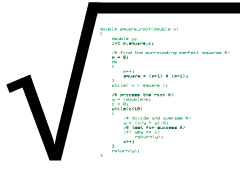# My Own Square Root FuntionFrom last week’s Lesson, I plowed into a BASIC programming book I worked on 35 years ago. (Yes, I’m old.) In it, substitute code was offered for commands not available in every version of BASIC. To appreciate this necessity, understand that back in those days computers weren’t file-to-file compatible, so translating programming language dialects was part of the job.

The algorithm presented in the book for calculating a square root was close, but misses the first step in the process: to find the closest known perfect square. This approach is one of many, and the one I selected to craft my own square root function. It’s based on this post on the math.com website.

Here are the steps for finding the square root of a value, X.

1. Find the nearest perfect square.
2. Divide the number, n, by the nearest perfect square.
3. Average the result of Step 2 and the perfect square.
4. If the result of Step 3 squared equals n, you’re done. Otherwise, continue to divide and average.

For my function, the first step is to generate perfect squares until you find one close to n. For example, to find the square root of 20, you could use 42 as 16 for the nearest perfect square.

The second step is to divide n by the perfect square: `20 / 4`, which equals 5.

The third step is to take the value from Step 2 and average it with the perfect square: `( 5 + 4 ) / 2`, which equals 4.5.

Fourth, square the result to see how close you are: `4.5 * 4.5 = 20.25`. If this value doesn’t equal the original value n (20), repeat the process using the new square root wannabe value:

`( 20 / 4.5 + 4.5 ) / 2 = 4.4722`, and `4.47222 = 20.00`

According to my calculator, the square root of 20 is 4.4721. The value 4.4722 is close enough. If not, you must repeat the process:

`( 20 / 4.4722 + 4.47225 ) / 2 = 4.4721`, and `4.47212 = 20.00`

Floating point values in C are precise only to a given number of digits. One problem I had was to determine when a wannabe square root values was good enough. My solution is presented in the following code:

### 2020_07_25-Lesson.c

```#include <stdio.h>

double square_root(double x)
{
double y;
int p,square,c;

/* find the surrounding perfect squares */
p = 0;
do
{
p++;
square = (p+1) * (p+1);
}
while( x > square );

/* process the root */
y = (double)p;
c = 0;
while(c<10)
{
/* divide and average */
y = (x/y + y)/2;
/* test for success */
if( y*y == x)
return(y);
c++;
}
return(y);
}

int main()
{
double pv,sr;

printf("Enter a positive value: ");
scanf("%lf",&pv);
if( pv <= 0 )
return(1);
sr = square_root(pv);
printf("The square root of %.0f is %f\n",
pv,
sr
);

return(0);
}```

The first thing the square_root() function does is calculate perfect squares. The do-while loop repeats until the square in integer `p` is just under argument `x`.

Second, a loop processes the expression `y = (x/y + y)/2`, where `y` is the wannabe square root value and `x` is the value passed to the function. a test is made `y*y == x` to determine whether the root is found. If so the function returns `y`. If not, it loops at most 10 more times to hone the value. It could probably loop fewer or more iterations, but without any way to compare significant digits in a floating point value, I choose 10 times.

As it stands, the code runs and generates values that compare solidly with the computer’s calculator app. Therefore, I am pleased.